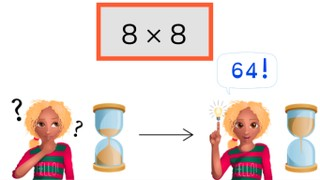Automaticity 8 times table

# Automaticity 8 times table

Students learn to memorize the 8 times table.8,000 schools use Gynzy92,000 teachers use Gynzy1,600,000 students use Gynzy

## General

The students memorize the 8 times table.

## Standards

CCSS.Math.Content.3.OA.C.7

## Learning objective

Students will be able to memorize the 8 times table and quickly give its products.

## Introduction

Discuss with the students which answers are in the 8 times table and which are not. Drag each of the answers to the correct column.

## Instruction

First have the students solve problems from the 8 times table within 5, 4, and 3 seconds. Then show the pile of cards. Explain that you are going to start the timer and remove the cover to show the first problem. Have the students solve the problem correctly, then drag the card aside to show the next problem. Try to solve all the problems within the time given by the timer.

Check whether the students have memorized the 8 times table with the following question:
- Why is it important to memorize the 8 times table?

## Quiz

The students test their memorization of the 8 times table with 3 timed exercises.

## Closing

Discuss once again the importance of memorizing the 8 times table. As a closing activity you play times table bingo. Spin the wheel and have the students solve the problems. When the answer is on the bingo card you drag a line over the number to cross it out. With three in a row either horizontally or vertically, you have a bingo.

## Teaching tips

Give students that have difficulty with memorizing the 8 times table a printed copy of the 8 times table to practice with. Have them practice in pairs using a timer to solve problems in shorter and shorter amounts of time.

## Instruction materials

You may print out a copy of the 8 times table.

### The online teaching platform for interactive whiteboards and displays in schools

• Save time building lessons

• Manage the classroom more efficiently

• Increase student engagement